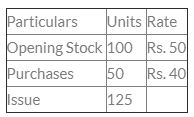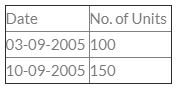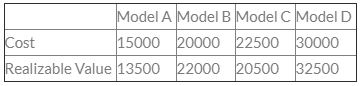Courses

# Test: Inventories - 1

## 30 Questions MCQ Test Fundamentals of Accounting for CA CPT | Test: Inventories - 1

Description
This mock test of Test: Inventories - 1 for CA Foundation helps you for every CA Foundation entrance exam. This contains 30 Multiple Choice Questions for CA Foundation Test: Inventories - 1 (mcq) to study with solutions a complete question bank. The solved questions answers in this Test: Inventories - 1 quiz give you a good mix of easy questions and tough questions. CA Foundation students definitely take this Test: Inventories - 1 exercise for a better result in the exam. You can find other Test: Inventories - 1 extra questions, long questions & short questions for CA Foundation on EduRev as well by searching above.
QUESTION: 1

Solution:
QUESTION: 2

Solution:
QUESTION: 3

### Purchases = Rs. 1,10,000, Return outward Rs. 10,000. Goods given away as charity = Rs. 1,500. Goods distributed as sample = Rs. 1,000. What is the amount of net purchases ?

Solution:
QUESTION: 4

Under Inflationary conditions, LIFO will lead to :

Solution:
QUESTION: 5

What is the amount of purchase when opening stock = Rs. 3,500 closing stock = Rs. 1,500, Cost of goods sold = Rs. 22,000.

Solution:
QUESTION: 6

If average stock is Rs. 20,000. Closing stock is Rs. 4,000 more than value of opening stock. Closing stock will be :

Solution:

let os be x , then cs will be 4000+x

average stock =x+x+4000/2=20000

os = 18000

cs = 22000

QUESTION: 7

AS – 2 Prescribes the use of which method of stock valuation?

Solution:
QUESTION: 8

Physical Inventory system is also known as :

Solution:
QUESTION: 9

What are the consequences of undervaluation of closing stock ?

Solution:
QUESTION: 10

When closing inventory will be overstated it will result in :

Solution:
QUESTION: 11

Opening Stock Rs. 40,000
Closing Stock Rs. 50,000
Purchases Rs. 5,50,000
Return outward Rs. 5,000
Return inward Rs. 20,000
Carriage inward Rs. 5,000
If gross profit is 20% of sales, the gross sales will be:

Solution:

Cost of goods sold(net)= Opening stock + Net purchases + direct exp. - closing stock

Substitute all values in the above formula,

Cost of goods sold(net) = 30000 + 545000 + 5000 - 40000

= INR 540000

Now question says profit is 20% of net sales which means profit is 1/5th of net sales. To be more clear  ,  1= profit and 5 = net sales in the above fraction...

So what is cost?

Cost = net sales - profit

=  5 - 1

= 4

Which we can say, that profit is 1/4th on cost of net sales...

So profit will be =   540000(cost of net sales) * 1/4th

= INR 135000

So now the Net sales will be = cost of net sales + profit

= 540000  +135000

Net sales                               = INR 675000

now gross sales will be   =  Net sales + returns

=  675000 + 20000

Gross sales                    =  INR 695000

QUESTION: 12

Find out value of Closing Stock :
Opening Stock Rs. 70,000
Purchase Rs. 4,16,000
Sales Rs. 5,22,000
Gross profit earned 25% of cost

Solution:

Accounting Equation to find out the cost of goods sold is :

Cost of Goods sold = Opening stock + Purchases - Closing Stock

Gross Profit earned is 25% on cost.

Let us assume cost is Rs.100

GP will @25% on cost i.e. Rs.25

Hence sales becomes cost of goods sold + Profit i.e. Rs.100 + Rs.25= Rs.125

Therefore Gross Profit on sales will be =  Gross Profit /  Sales * 100

Profit on sales = Rs.25 / Rs.125 * 100 i.e 20% on Sales

In the given problem Sales is Rs. 522000

Hence Gross Profit will be 20% of Rs.522000 i.e. Rs.104400

Cost of Goods Sold = Rs.522000 - Rs.104400

Cost of goods sold = Rs.417600

Therefore

Rs.417600 = Rs.70000 + Rs.416000 - Closing stock

Closing stock = Rs.486000 - Rs.417600

Closing Stock = Rs.68400

QUESTION: 13

Opening stock    Rs. 10,000
Purchases         Rs. 1,10,000
Closing Stock    Rs. 20,000
Find out total sales if profit margin is 30% on cost of sales :

Solution:

COGS=OPENING STOCK +PURCHASE- CLOSING STOCK

=10000+110000-20000=100000 sales =cost +profit =100000+30000 =130000

QUESTION: 14

Inventories are assets :

Solution:
QUESTION: 15

Damaged inventory should be valued at:

Solution:
QUESTION: 16

The revised Accounting Standard-2 (Valuation of Inventories) permits which of the following method for computation of cost of Inventory?

Solution:
QUESTION: 17

Opening Stock = Rs. 6,000
Closing Stock = Rs. 8,000
Cost of Goods Sold = Rs. 87,000
Calculate the value of Purchases ?

Solution:
QUESTION: 18

If cost of goods sold is Rs. 80,700, opening stock Rs.5,800 and closing stock Rs. 6,000, then the amount of purchase will be :-

Solution:
QUESTION: 19

The books of T Ltd. revealed the following information:
Opening inventory                                   Rs.6,00,000
Purchases during the year 2010-2011       Rs.34,00,000
Sales during the year 2010-2011              Rs.48,00,000
On March 31, 2011, the value of inventory as per physical Inventory-taking was Rs. 3,25,000. The company’s gross profit on sales has remained constant at 25%. The management of the company suspects that some inventory might have been pilfered by a new employee. What is the estimated cost of missing inventory?

Solution:
QUESTION: 20

The total cost of gods available for sale with a company during the current years is Rs. 12,00,000 and the total sales during the period are Rs. 13,00,000. If the gross profit margin of the company is 331 /3% on cost, the closing inventory during the current year is

Solution:
QUESTION: 21

Calculate Closing Stock using FIFO Method:Solution:
QUESTION: 22

Goods purchased Rs. 1,00,000. Sales Rs. 90,000. Margin 20% on cost. Closing Inventory =?

Solution:
QUESTION: 23

On April 07,2011, i.e., a week after the end of the accounting year 2010-11, a company undertook physical Inventory verification. The value of Inventory as per physical Inventory verification was found to be Rs. 35,000.
The following details pertaining to the period April 01, 2011 to April 07,2011 are given:
I. Goods costing Rs. 5,000 were sold during the week.
II.Goods received from consignor amounting to Rs. 4,000 included in the value of Inventory.
III.Goods earlier purchased but returned during the period amounted to Rs. 1,000..
IV.Goods earlier purchased and accounted but not received Rs. 6,000.
After considering the above, the value of Inventories held as on March 31,2011 was

Solution:
QUESTION: 24

Consider the following information pertaining to G & Sons as on March 31, 2011:
Opening inventory                              Rs.15,00,000
Purchases during the year 2010-11     Rs.45,00,000
Sales during the year 2010-11            Rs.50,00,000
As per physical inventory taken on March 31, 2011 the closing inventory was Rs.20,90,000. Gross profit on sales has remained constant at 25%. The management of the firm suspects that some inventory might have been taken away by a new employee. The estimated cost of missing inventory on the close of the financial year and the cost of goods sold during the year, respectively are

Solution:
QUESTION: 25

C Ltd. recorded the following information as on March 31,2011:
Stock as on April 01, 2010   Rs. 80,000
Purchases                          Rs.1,60,000
Sales                                 Rs.2,00,000
It is noticed that goods worth Rs.30,000 were destroyed due to fire. Against this, the insurance company accepted a claim of Rs. 20,000.
The company sells goods at cost plus 33 1/3%. The value of closing inventory, after taking into account the above transactions is,

Solution:
QUESTION: 26

Calculate the value of purchase through following details :
Opening Stock Rs. 20,000
Sales Rs. 1,50,000
Gross profit Margin Rs. 20% of sales
Closing Stock Rs. 30,000

Solution:
QUESTION: 27

D Company, a dealer in cosmetics, records its inventory under first-in-first-out method, so as to minimize accumulation of outdated Inventory. The opening stock as on September 01, 2011 is 150 units at the rate of Rs. 20 per unit. The purchases and sales made during the month are:
Purchases:Sales:With effect from September 01,2011, the company decided to change the method of inventory valuation from the FIFO method to LIFO method. The change in the value of inventory as on September 30,2011 consequent upon the change in the method of valuation is

Solution:
QUESTION: 28

Average Inventory = Rs. 12,000. Closing stock is Rs. 3,000 more than opening stock. The value of closing Inventory = _________.

Solution:
QUESTION: 29

X & Company, a furniture dealer, due to some business problem could take physical stock taking on April 20 and arrived at the cost at Rs.5,25,000. Between April 01 and  April 20 firm even though purchased goods worth Rs. 3,25,000 including credit purchases of Rs. 75,000 only goods costing Rs. 50,000 was not actually received before April 20. Cost of goods held at godown on March 31 was:

Solution:
QUESTION: 30

Four Washing Machines are in stock with a dealerFind out the value of stock for balance sheet as per AS-2

Solution: